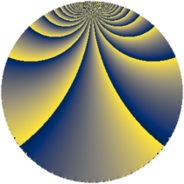# Properties

 Label 684.3.baLevel $684$ Weight $3$ Character orbit 684.ba Rep. character $\chi_{684}(107,\cdot)$ Character field $\Q(\zeta_{6})$ Dimension $160$ Sturm bound $360$

# Related objects

## Defining parameters

 Level: $$N$$ $$=$$ $$684 = 2^{2} \cdot 3^{2} \cdot 19$$ Weight: $$k$$ $$=$$ $$3$$ Character orbit: $$[\chi]$$ $$=$$ 684.ba (of order $$6$$ and degree $$2$$) Character conductor: $$\operatorname{cond}(\chi)$$ $$=$$ $$228$$ Character field: $$\Q(\zeta_{6})$$ Sturm bound: $$360$$

## Dimensions

The following table gives the dimensions of various subspaces of $$M_{3}(684, [\chi])$$.

Total New Old
Modular forms 496 160 336
Cusp forms 464 160 304
Eisenstein series 32 0 32

## Trace form

 $$160q - 4q^{4} + O(q^{10})$$ $$160q - 4q^{4} - 60q^{10} + 48q^{13} - 28q^{16} + 400q^{25} + 12q^{34} - 1216q^{49} - 240q^{52} + 128q^{58} + 16q^{61} + 464q^{64} - 144q^{70} + 240q^{73} - 236q^{76} - 284q^{82} + 352q^{85} + O(q^{100})$$

## Decomposition of $$S_{3}^{\mathrm{new}}(684, [\chi])$$ into newform subspaces

The newforms in this space have not yet been added to the LMFDB.

## Decomposition of $$S_{3}^{\mathrm{old}}(684, [\chi])$$ into lower level spaces

$$S_{3}^{\mathrm{old}}(684, [\chi]) \cong$$ $$S_{3}^{\mathrm{new}}(228, [\chi])$$$$^{\oplus 2}$$